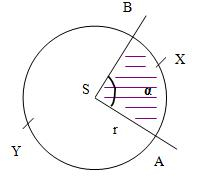# Corresponding 59063

Calculate the radius and area of the circular segment if the center angle = 106° and the length of the corresponding circular arc is l = 52 cm.

r =  28.1074 cm
S =  730.7915 cm2

### Step-by-step explanation:Did you find an error or inaccuracy? Feel free to write us. Thank you!

Tips for related online calculators
Need help calculating sum, simplifying, or multiplying fractions? Try our fraction calculator.

#### Grade of the word problem:

We encourage you to watch this tutorial video on this math problem: